## Capacitance of Two Wire Line:

Consider a Capacitance of Two Wire Line shown in Fig. 3.3 excited from a single-phase source. The Capacitance of Two Wire Line develops equal and opposite sinusoidal charges on the two conductors which can be represented as phasors qa and qb so that qa = – qb.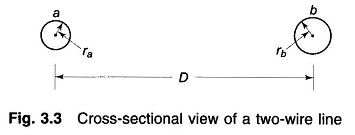The potential difference Vab can be written in terms of the contributions made by qa and qb by use of Eq. (3.2) with associated assumptions (i.e. D/r is large and ground is far away). Thus,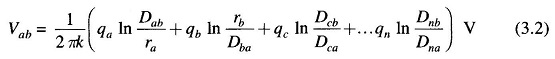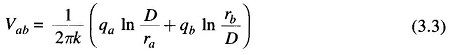Since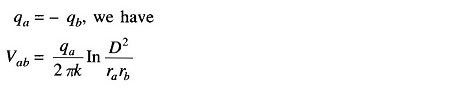The line capacitance Cab is then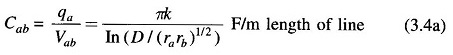or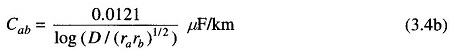If ra = rb = r,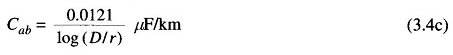The associated line charging current is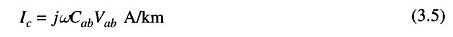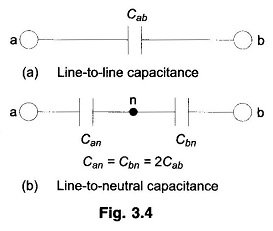As shown in Figs. 3.4 (a) and (b) the line-to-line capacitance can be equivalently considered as two equal capacitances is series. The voltage across the lines divides equally between the capacitances such that the neutral point n is at the ground potential. The capacitance of each line to neutral is then given by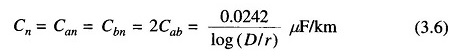#### The assumptions inherent in the above derivation are:

1. The charge on the surface of each conductor is assumed to be uniformly distributed, but this is strictly not correct.

If non-uniformity of charge distribution is taken into account, then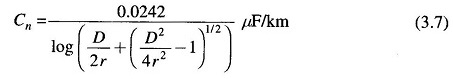If D/2r ≫ 1, the above expression reduces to that of Eq. (3.6) and the error caused by the assumption of uniform charge distribution is negligible.

2. The cross-section of both the conductors is assumed to be circular, while in actual practice stranded conductors are used. The use of the radius of the circumscribing circle for a stranded conductor causes insignificant error.

Scroll to Top